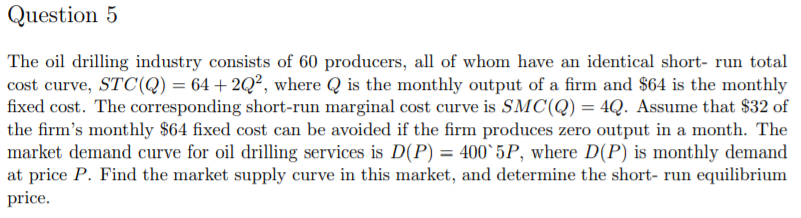# Please answer me in detail. Thank you. Market demand curve is D(P)=400-5P. The oil drilling industry consists of 60 pro...Market demand curve is D(P)=400-5P.

The oil drilling industry consists of 60 producers, all of whom have an identical short- run total cost curve, STC(Q) = 64 + 2Q2, where Q is the monthly output of a firm and \$64 is the monthly fixed cost. The corresponding short-run marginal cost curve is SMC(Q) 4Q. Assume that \$32 of the firm's monthly \$64 fixed cost can be avoided if the firm produces zero output in a month. The market demand curve for oil drilling services is D(P)-400 5P, where D(P) is monthly demand at price P. Find the market supply curve in this market, and determine the short- run equilibrium price

P= SMC= 4Q

Q= P/4

This is the firm's supply function.

Market supply curve= 60*Q= 60*P/4= 15P

At equilibrium, supply= demand

15P= 400-5P

20P= 400

P= 20

##### Add Answer of: Please answer me in detail. Thank you. Market demand curve is D(P)=400-5P. The oil drilling industry consists of 60 pro...
More Homework Help Questions Additional questions in this topic.